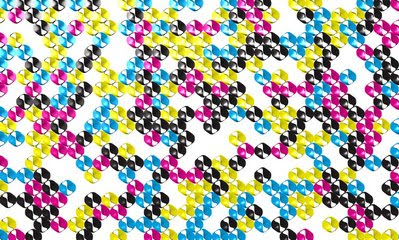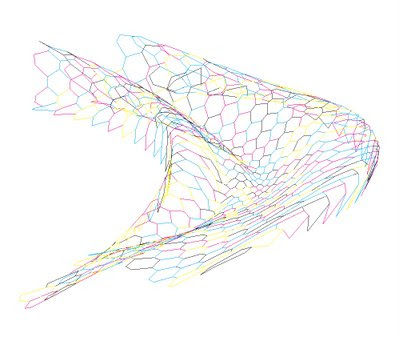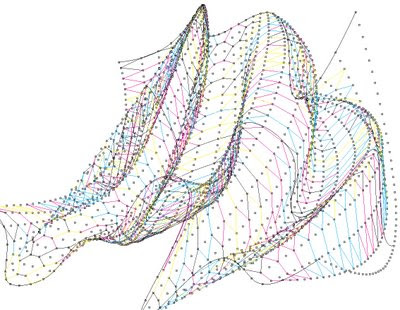## Tuesday, March 13, 2007

### 070312_HoneyComb (re-scripting)TILING
A plane-filling arrangement of plane figures or its generalization to higher dimensions. Formally, a tiling is a collection of disjoint open sets, the closures of which cover the plane. Given a single tile, the so-called first corona is the set of all tiles that have a common boundary point with the tile (including the original tile itself).

Wang's conjecture (1961) stated that if a set of tiles tiled the plane, then they could always be arranged to do so periodically. A periodic tiling of the plane by polygons or space by polyhedra is called a tessellation. The conjecture was refuted in 1966 when R. Berger showed that an aperiodic set of 20426 tiles exists. By 1971, R. Robinson had reduced the number to six and, in 1974, R. Penrose discovered an aperiodic set (when color-matching rules are included) of two tiles: the so-called Penrose tiles. It is not known if there is a single aperiodic tile.
(ie mathworld.com)HONEY COMB
As part of a re-visiting codes series -most often following students requirments- I went back to one of my very first rhinoscript code generating Honeycomb cells onto a host nurbs surface and rewrote it... mainly shorter, for sure much faster and somehow within a concern of a certain "elegance" -to quote the latest trend of fitness criteria accoding to Ali Rahim's latest issue of AD magasine!- as an exercice within the exercice...

Tips and tricks:
The generation of the honeycomb cells within rhinoscript is now defined by one unique conditional statment using many booleans operations.
If (i>1 And j>1) And ( ( ((i-1)Mod 2) And (((j-2)Mod 4)= 0) ) Or ( (((i-1)Mod 2)=0) And ((j Mod 4)=0) ) Or ( (((i-1)Mod 2)=0) And (((j-1)Mod 4)=0) ) Or ( (((i-1)Mod 2)) And (((j+1)Mod 4)=0) ) )HONEYCOMB GEOMETRY: (ie wikipedia.org)
The axes of honeycomb cells are always quasi-horizontal, and the non-angled rows of honeycomb cells are always horizontally (not vertically) aligned. Thus, each cell has two vertical walls, with "floors" and "ceilings" composed of two angled walls. The cells slope slightly upwards, between 9 and 14 degrees, towards the open ends.

There are two possible explanations for the reason that honeycomb is composed of hexagons, rather than any other shape. One, given by Jan Brożek, is that the hexagon tiles the plane with minimal surface area. Thus a hexagonal structure uses the least material to create a lattice of cells with a given volume. Another, given by D'Arcy Wentworth Thompson, is that the shape simply results from the process of individual bees putting cells together: somewhat analogous to the boundary shapes created in a field of soap bubbles. In support of this he notes that queen cells, which are constructed singly, are irregular and lumpy with no apparent attempt at efficiency.

It is likely that the honey bee constructs the honeycomb based on instinct, and the prevailing theory of biology is that the appearance of such efficient shapes in nature is a result of natural selection.

The closed ends of the honeycomb cells are also an example of geometric efficiency, albeit three-dimensional and little-noticed. The ends are trihedral (i.e., composed of three planes) pyramidal in shape, with the dihedral angles of all adjacent surfaces measuring 120°, the angle that minimizes surface area for a given volume. (The angle formed by the edges at the pyramidal apex is approximately 109° 28' 16" (= 180° - arccos(1/3)).)

The shape of the cells is such that two opposing honeycomb layers nest into each other, with each facet of the closed ends being shared by opposing cells.

Individual cells do not, of course, show this geometrical perfection: in a regular comb, there are deviations of a few percent from the "perfect" hexagonal shape. In transition zones between the larger cells of drone comb and the smaller cells of worker comb, or when the bees encounter obstacles, the shapes are often distorted.

In 1965, László Fejes Tóth discovered that the trihedral pyramidal shape (which is composed of three rhombi) used by the honey bee is not the theoretically optimal three-dimensional geometry. A cell end composed of two hexagons and two smaller rhombuses would actually be .035% (or approximately 1 part per 2850) more efficient. This difference is too minute to measure on an actual honeycomb, and irrelevant to the hive economy in terms of efficient use of wax, considering that wild comb varies considerably from any human notion of "ideal" geometry.

Labels: , ,